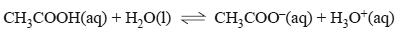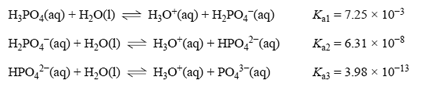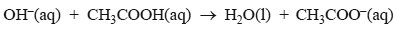Acid/base equilibria 2013 VCE 1) Which one of the following solutions has the highest pH? A. 0.01 M HCOOH B. 1.0 M HCOOH C. 0.01 M CH3COOH D. 1.0 M CH3COOH Solution Mathematically validate the answer2) The ionisation of ethanoic acid can be represented by the equationThe percentage ionisation of ethanoic acid is greatest in a A. 50 mL 1.0 M CH3COOH solution. B. 50 mL 0.1 M CH3COOH solution. C. 100 mL 0.1 M CH3COOH solution. D. 100 mL 0.01 M CH3COOH solution. Solution 3) Phosphoric acid is present in cola-flavoured soft drinks and has been linked to decreased bone density. It is a triprotic acid with the following Ka values at 25 °C.To determine the approximate pH of a 0.1 M phosphoric acid solution, a student should use the value of A. Ka1 only B. Ka3 only C. Ka1 × Ka3 only D. Ka1 × Ka2 × Ka3 Solution4) A 20.00 mL aliquot of 0.200 M CH3COOH (ethanoic acid) is titrated with 0.150 M NaOH. The equation for the reaction between the ethanoic acid and NaOH solution is represented below.a) What volume of the NaOH solution is required to completely react with the ethanoic acid? Solutionb) Write an expression for the acidity constant of ethanoic acid. Solutionc) Calculate the pH of the 0.200 M ethanoic acid solution before any NaOH solution has been added. Assume that the equilibrium concentration of the ethanoic acid is 0.200 M. Solutiond) Consider the point in the titration where the volume of NaOH added is exactly half that required for complete neutralisation. What is the relationship between the concentration of H3O+and Ka at this point ? Solution